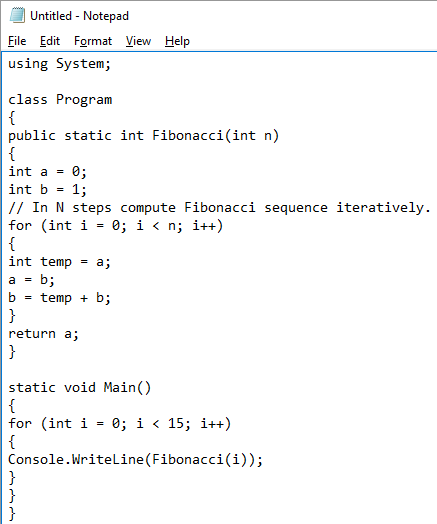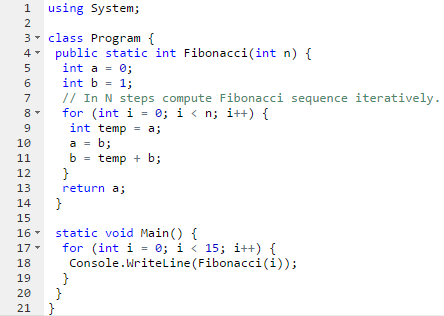# Format your code when you are posting it online

Warning, semirant incoming

There are multiple ways to post code online. And most of them should be banned!

Sample code shown in here is copied+pasted from Dot Net Perls

## The worst(tm) possible way to paste code

This award goes to anyone that takes a screenshot from badly formatted code and pastes that screenshot to forum/blog/IM.• It is hard to read the code (neither formatting nor folding)
• It is hard to copy+paste code
• It is hard to directly call that code

## Bad way to paste code

This award goes to anyone that takes a screenshot from formatted code and pastes that screenshot to forum/blog/IM.• It is hard to copy+paste code
• It is hard to directly call that code

## Horrible way to paste code

This award goes to anyone that just dumps code to plain textinput. Output is then assumed to be regular text.

using System;

class Program { public static int Fibonacci(int n) { int a = 0; int b = 1; // In N steps compute Fibonacci sequence iteratively. for (int i = 0; i < n; i++) { int temp = a; a = b; b = temp + b; } return a; }

static void Main() { for (int i = 0; i < 15; i++) { Console.WriteLine(Fibonacci(i)); } } }

• It is hard to read the code (neither formatting nor folding)
• It is hard to directly call that code

## Mediocre way to paste code

This award goes to anyone that pastes code with folding but does NOT use color coding.

``````using System;

class Program
{
public static int Fibonacci(int n)
{
int a = 0;
int b = 1;
// In N steps compute Fibonacci sequence iteratively.
for (int i = 0; i < n; i++)
{
int temp = a;
a = b;
b = temp + b;
}
return a;
}

static void Main()
{
for (int i = 0; i < 15; i++)
{
Console.WriteLine(Fibonacci(i));
}
}
}
``````

• It is not easy to read the code
• It is hard to directly call that code

## Good way to paste code online

This award goes to anyone that pastes code with proper formatting and folding to forum/blog/IM.

``````using System;

class Program
{
public static int Fibonacci(int n)
{
int a = 0;
int b = 1;
// In N steps compute Fibonacci sequence iteratively.
for (int i = 0; i < n; i++)
{
int temp = a;
a = b;
b = temp + b;
}
return a;
}

static void Main()
{
for (int i = 0; i < 15; i++)
{
Console.WriteLine(Fibonacci(i));
}
}
}
``````

• It is hard to directly call that code

## Better way to paste code online

This award goes to anyone that pastes code to gist (or similar service) and links that to the post.